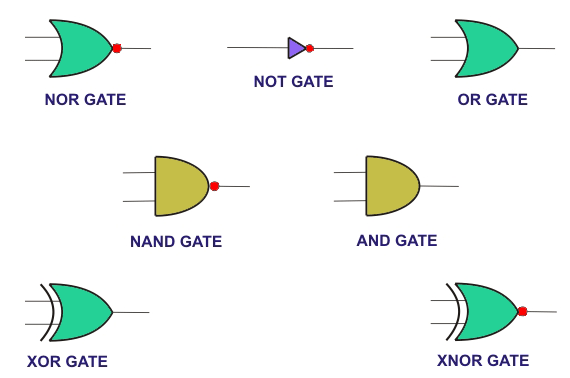# Digital Logic Gates

Digital logic gate is an electronic component which results in a particular output after implementing its logic on the input signals. These serve as the basic building blocks of any digital system irrespective of its complexity. Digital logic gates (Figure 1) can be categorized into two types.

## Basic Gates

NOT gate has one input and one output, where the output will always be the inversion (i.e. negation) of the input. This means that if the input is zero, then output will be one and vice-versa.
AND gate can have multiple inputs, while its output is restricted to be a single one. Here the output will be high only if all the inputs are high; for any other case the output will be low.

OR gate is a multi-input, single-output gate which has its output equal to logic ‘zero’ when all the inputs to the gate are low. Further for all other remaining combination of inputs, where in at least one of the inputs will be high, the output will be logically high.
NOT, AND and OR gates are the preliminary gates used to realize any Boolean expression. Thus these are called basic gates.## Universal Gates

AND gate in conjunction with a NOT gate yields a NAND gate which is a multi-in, single-out logic gate. This gate has its output low only if all its inputs are at logical high level. On the other hand for any other input combination the output of the gate will be high.
NOR gate is a combination of OR gate with a NOT gate. Here the output will be at the logic level ‘one’ only when all the inputs to the gate are at low state; all other combination of inputs result in logical zero at the gate output.
NAND and NOR gates are considered to be universal gates because any logical function can be realized just by using these two gates.

Apart from these, two more gates viz., XOR and XNOR are extensively used. XOR gate can have any number of inputs while its output is restricted to a single line. For a two-input XOR gate, the output is high only if the inputs are non-identical, else (if identical) the output will be low. For example if the two inputs are 0 and 1, then the output will be 1; else if the inputs are 0 and 0 (or 1 and 1) then the output will be 0. XOR in conjunction with a NOT gate yields XNOR gate whose working is complementary to that of XOR. This means that the output of the XNOR gate is high for identical inputs and low for non-identical inputs.
Digital logic gates can be realized using transistors, diodes and other basic electronic components. However commercially they are available in the form of integrated circuits (ICs) working either on Transistor Transistor Logic (TTL) or on Complementary Metal Oxide Silicon (CMOS) technology.

These devices operate on either ‘positive’ or ‘negative’ logic. In positive logic devices, a high voltage level will be considered to be logic ‘one’ while the zero voltage represents the logic state ‘zero’. On the other hand if the device operates on ‘negative logic’, then the high voltage will be considered to be logic zero while the low voltage will be considered to be logic high. Any voltage between 2 to 5 V is considered to be high in case of TTL while in CMOS the range is from 3 to 18 V. Similarly any voltage below 0.8 V is considered to be logic ‘zero’ in case of TTL while for CMOS it is below 1.5 V. Appearance of any voltage in between the specified range at the input of the digital logic gates will cause it to malfunction. Inorder to overcome this, these devices are expected to be immune to external noise.

Want To Learn Faster? 🎓
Get electrical articles delivered to your inbox every week.
No credit card required—it’s 100% free.